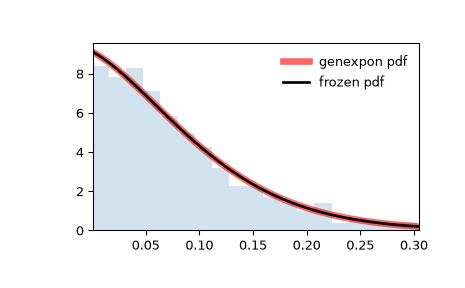# scipy.stats.genexpon¶

scipy.stats.genexpon = <scipy.stats._continuous_distns.genexpon_gen object>[source]

A generalized exponential continuous random variable.

As an instance of the rv_continuous class, genexpon object inherits from it a collection of generic methods (see below for the full list), and completes them with details specific for this particular distribution.

Notes

The probability density function for genexpon is:

$f(x, a, b, c) = (a + b (1 - \exp(-c x))) \exp(-a x - b x + \frac{b}{c} (1-\exp(-c x)))$

for $$x \ge 0$$, $$a, b, c > 0$$.

genexpon takes $$a$$, $$b$$ and $$c$$ as shape parameters.

The probability density above is defined in the “standardized” form. To shift and/or scale the distribution use the loc and scale parameters. Specifically, genexpon.pdf(x, a, b, c, loc, scale) is identically equivalent to genexpon.pdf(y, a, b, c) / scale with y = (x - loc) / scale. Note that shifting the location of a distribution does not make it a “noncentral” distribution; noncentral generalizations of some distributions are available in separate classes.

References

H.K. Ryu, “An Extension of Marshall and Olkin’s Bivariate Exponential Distribution”, Journal of the American Statistical Association, 1993.

N. Balakrishnan, “The Exponential Distribution: Theory, Methods and Applications”, Asit P. Basu.

Examples

>>> from scipy.stats import genexpon
>>> import matplotlib.pyplot as plt
>>> fig, ax = plt.subplots(1, 1)


Calculate the first four moments:

>>> a, b, c = 9.13, 16.2, 3.28
>>> mean, var, skew, kurt = genexpon.stats(a, b, c, moments='mvsk')


Display the probability density function (pdf):

>>> x = np.linspace(genexpon.ppf(0.01, a, b, c),
...                 genexpon.ppf(0.99, a, b, c), 100)
>>> ax.plot(x, genexpon.pdf(x, a, b, c),
...        'r-', lw=5, alpha=0.6, label='genexpon pdf')


Alternatively, the distribution object can be called (as a function) to fix the shape, location and scale parameters. This returns a “frozen” RV object holding the given parameters fixed.

Freeze the distribution and display the frozen pdf:

>>> rv = genexpon(a, b, c)
>>> ax.plot(x, rv.pdf(x), 'k-', lw=2, label='frozen pdf')


Check accuracy of cdf and ppf:

>>> vals = genexpon.ppf([0.001, 0.5, 0.999], a, b, c)
>>> np.allclose([0.001, 0.5, 0.999], genexpon.cdf(vals, a, b, c))
True


Generate random numbers:

>>> r = genexpon.rvs(a, b, c, size=1000)


And compare the histogram:

>>> ax.hist(r, density=True, histtype='stepfilled', alpha=0.2)
>>> ax.legend(loc='best', frameon=False)
>>> plt.show()Methods

 rvs(a, b, c, loc=0, scale=1, size=1, random_state=None) Random variates. pdf(x, a, b, c, loc=0, scale=1) Probability density function. logpdf(x, a, b, c, loc=0, scale=1) Log of the probability density function. cdf(x, a, b, c, loc=0, scale=1) Cumulative distribution function. logcdf(x, a, b, c, loc=0, scale=1) Log of the cumulative distribution function. sf(x, a, b, c, loc=0, scale=1) Survival function (also defined as 1 - cdf, but sf is sometimes more accurate). logsf(x, a, b, c, loc=0, scale=1) Log of the survival function. ppf(q, a, b, c, loc=0, scale=1) Percent point function (inverse of cdf — percentiles). isf(q, a, b, c, loc=0, scale=1) Inverse survival function (inverse of sf). moment(n, a, b, c, loc=0, scale=1) Non-central moment of order n stats(a, b, c, loc=0, scale=1, moments=’mv’) Mean(‘m’), variance(‘v’), skew(‘s’), and/or kurtosis(‘k’). entropy(a, b, c, loc=0, scale=1) (Differential) entropy of the RV. fit(data) Parameter estimates for generic data. See scipy.stats.rv_continuous.fit for detailed documentation of the keyword arguments. expect(func, args=(a, b, c), loc=0, scale=1, lb=None, ub=None, conditional=False, **kwds) Expected value of a function (of one argument) with respect to the distribution. median(a, b, c, loc=0, scale=1) Median of the distribution. mean(a, b, c, loc=0, scale=1) Mean of the distribution. var(a, b, c, loc=0, scale=1) Variance of the distribution. std(a, b, c, loc=0, scale=1) Standard deviation of the distribution. interval(alpha, a, b, c, loc=0, scale=1) Endpoints of the range that contains fraction alpha [0, 1] of the distribution